### Home > A2C > Chapter Ch9 > Lesson 9.1.1 > Problem9-8

9-8.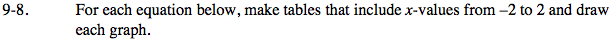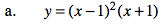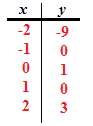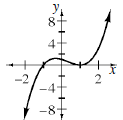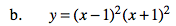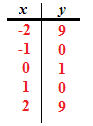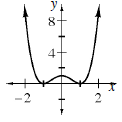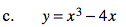Use the same method as in parts (a) and (b).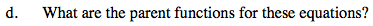What is the highest-valued exponent?

The parent function is y = x3.
y = (x − 1)2(x + 1)
y = (x2 − 2x + 1)(x + 1)
y = x3x2x + 1